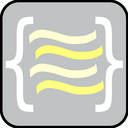reallyba
•  1,704,541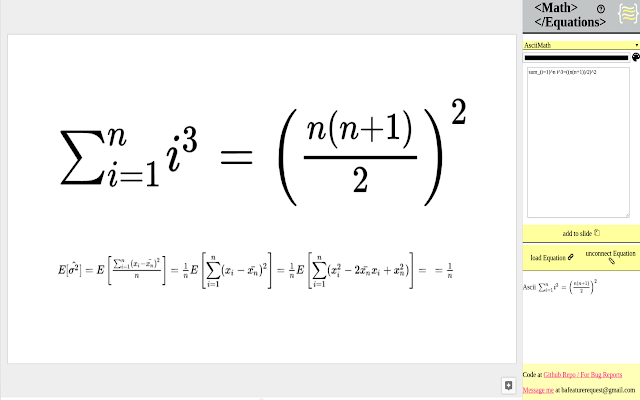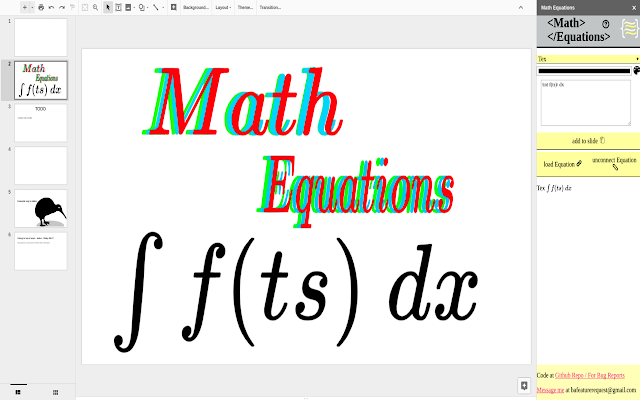Overview
Convert Latex, AsciiMath and MathML equations to an image.
```Math Equations allows you to take your typeset languages and convert them to images to use inside of your slideshow.  Other features include the ability to reload a equation image and make changes and re upload to your presentation.

Recent changes added the math equations data into the alt text, which you can see by clicking using control + alt + y.

Supported Languages are
-Latex
-AsciiMath
-MathML

Other Features
-Font Color
-Font Size

If you have any problems just send my a message at
bafeaturerequest@gmail.com
Code can be located at

If this extension is interested you can check out my other extension that allows you to create math equations on any site.

Examples
Tex
--valid Tex equation

x^n + y^n = z^n

Tex type uses inline notation.  Which restricts what you can add in your equation.

Add \[ ]\ will cause a error at the current iteration.
Also many formatting tags and the use of usepackage will cause a error. Some of these feature i will include after i build a separate window to work on larger equations.

MathML
--Valid MathML

<mi>&#x3C0;</mi>
<mo>&#x2062;</mo>
<msup>
<mi>r</mi>
<mn>2</mn>
</msup>

MathML doesn't need to have a [itex] tag to be a valid equation.

AsciiMath
--Valid AsciiMath

sum_(i=1)^n i^3=((n(n+1))/2)^2

```
Reviews
Language:
Sort by: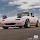A User of Math Equations
July 5, 2020
very useful
Yes
No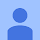A User of Math Equations
July 1, 2020
Extremely useful, saves a lot of time when creating presentations and is extremely intuitive to use.
Yes
No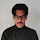A User of Math Equations
June 19, 2020
Very useful. You can install the addon, but when you try to use it, you realize that for some reason you can't activate the addon.
Yes
No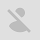A user of Math Equations
June 22, 2020
I have difficulty installing Math add-on.A User of Math Equations
May 13, 2020
it wouldnt tell me if i could put any symbols like division signs and stuff.It only like did stuff for like higher grades i am only in fourth grade and in the covid-19 pandemic so its harder to do stuff omline
Yes
NoA user of Math Equations
May 15, 2020
If you type the word "divide" you get the division symbol If you use "/" the equation is shown as a fraction.A User of Math Equations
March 26, 2020
Yes
NoA user of Math Equations
May 4, 2020
boi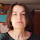A User of Math Equations
March 25, 2020
If you know LateX basics, this is great!
Yes
NoA User of Math Equations
March 10, 2020
Essential for math teachers using Google Slides to present lessons. A list of supported TeX syntax would be helpful.
Yes
No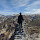A User of Math Equations
March 1, 2020
This is great, thank you so much :D
Yes
No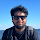A User of Math Equations
February 29, 2020
Just the thing I needed. Thank you so much!
Yes
NoA User of Math Equations
February 7, 2020
there are no instructions id give it 0 stars if i could
Yes
NoA user of Math Equations
July 7, 2020
All the add-on apps are saying unavailable for install, install disabled by admin. why?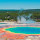A user of Math Equations
April 17, 2020
so true i agreeA user of Math Equations
March 1, 2020